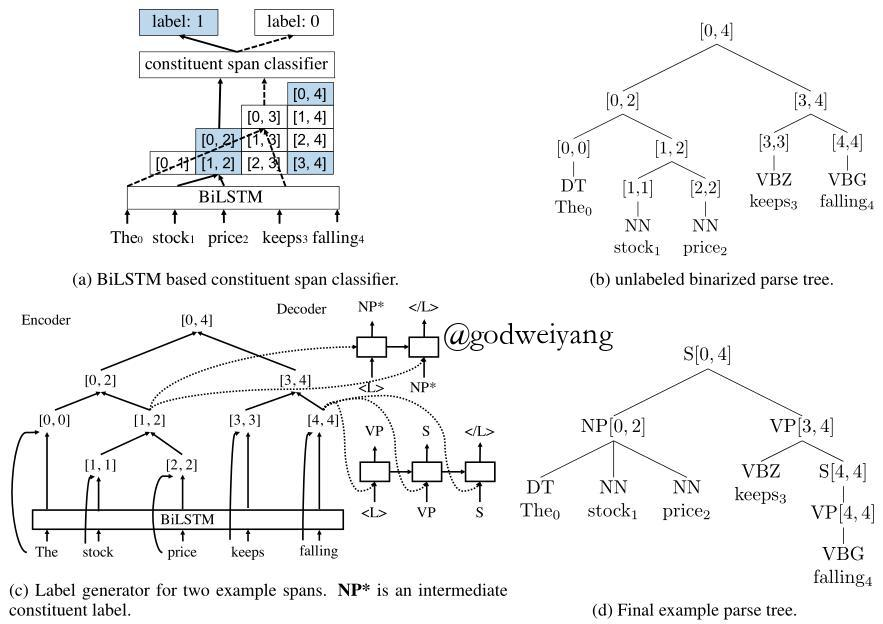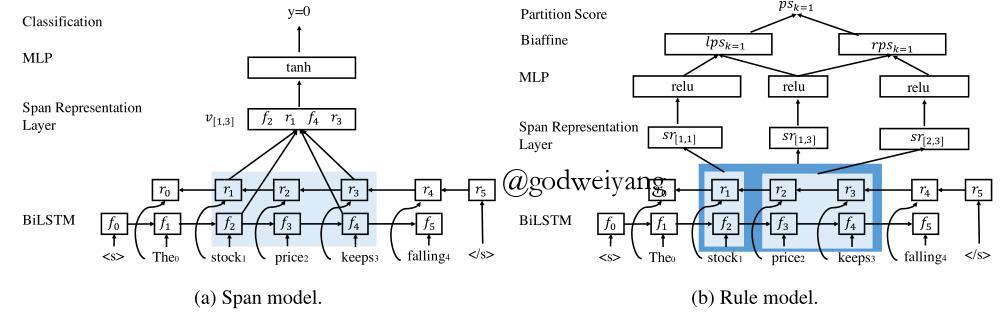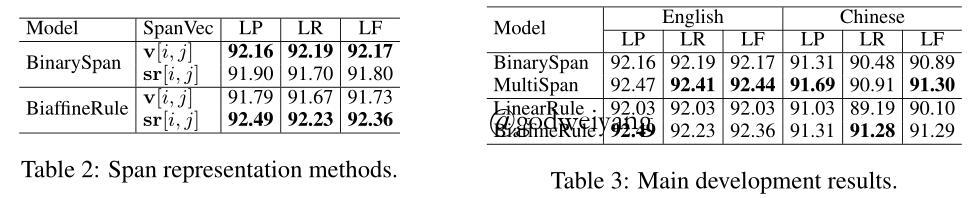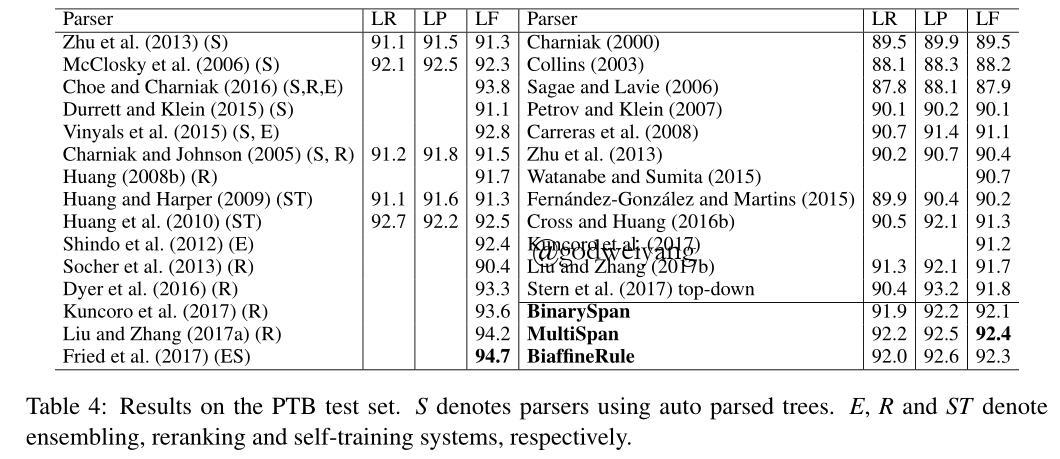Two Local Models for Neural Constituent Parsing# 模型

## Span模型

$v[i, j] = [f_{i + 1}; r_i; f_{j + 1}; r_j]$

$\begin{array}{l}o[i,j] = \tanh ({W_o}v[i,j] + {b_o})\\\\u[i,j] = {W_u}o[i,j] + {b_u}\\\\P({Y_{[i,j]}}|S,\Theta ) = softmax(u[i,j])\end{array}$

$P(r|S, \Theta) = P(Y_{[i, k]} = 1|S, \Theta)P(Y_{[k + 1, j]} = 1|S, \Theta)$

## Rule模型

span模型是训练出每个span属于标准树的概率，然后预测时计算出产生式的概率。而rule模型就直接训练每个产生式的概率，对于span$(i, j)$，假设它的split为k的分数为$ps_k$，那么产生式的概率就为：
$P([i, j] \to [i, k][k + 1, j] | S, \Theta) = \frac{\exp (ps_k)}{\sum\nolimits_{k’ = i}^{j - 1} {\exp (p{s_{k’}})} }$

$s[i, j] = [f_{j + 1} - f_i; r_i - r_{j + 1}]$

$sr[i, j] = [s[0, i - 1]; s[i, j]; s[j + 1, n - 1]]$

$r[i, j] = \phi (W_r^M sr[i, j] + b_r^M)$

$lps_k = {[r[i,j];1]^T}{W_{pl}}[r[i,k];1]$

$ps_k = lps_k + rps_k$

## 联合训练span模型和rule模型的span得分计算如下图所示：# 实验结果# 总结

• 二分类span模型：预测每个span属于标准树的概率，测试阶段使用CKY算法解码，通过span正确的概率计算得到产生式概率。
• 多分类span模型：在二分类的基础上，预测每个span是每个label的概率，最后求和得到它属于标准树的概率，测试过程和二分类相同。
• 线性组合rule模型：通过split的得分直接预测每个产生式的概率，采用线性组合计算概率，解码采用CKY算法。
• 双仿射rule模型：除了概率计算采用双仿射，其他部分和线性组合rule模型完全相同。

转载规则

《Two Local Models for Neural Constituent Parsing》 韦阳 采用 知识共享署名 4.0 国际许可协议 进行许可。
上一篇Meta Multi-Task Learning for Sequence Modeling

2018-10-25Linear-Time Constituency Parsing with RNNs and Dynamic Programming

2018-10-15
目录